### SOLUTIONS TO INTEGRATION BY PARTIAL FRACTIONS

SOLUTION 1 : Integrate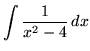. Factor and decompose into partial fractions, getting(After getting a common denominator, adding fractions, and equating numerators, it follows that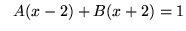;
let;
let.)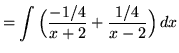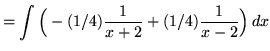(Recall that.).

SOLUTION 2 : Integrate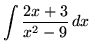. Factor and decompose into partial fractions, getting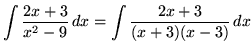(After getting a common denominator, adding fractions, and equating numerators, it follows that;
let;
let.)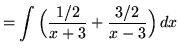.

SOLUTION 3 : Integrate. Factor and decompose into partial fractions, getting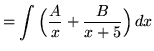(After getting a common denominator, adding fractions, and equating numerators, it follows that;
let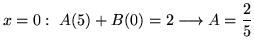;
let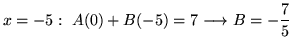.)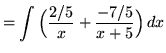.

SOLUTION 4 : Integrate. Because the degree of the numerator is not less than the degree of the denominator, we must first do polynomial division. Then factor and decompose into partial fractions, getting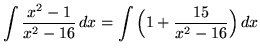(After getting a common denominator, adding fractions, and equating numerators, it follows that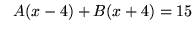;
let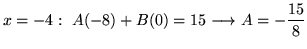;
let.)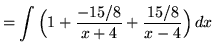(Recall that.).

SOLUTION 5 : Integrate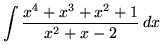. Because the degree of the numerator is not less than the degree of the denominator, we must first do polynomial division. Then factor and decompose into partial fractions, getting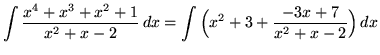(After getting a common denominator, adding fractions, and equating numerators, it follows that;
let;
let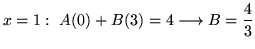.)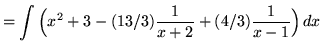.

SOLUTION 6 : Integrate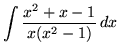. Factor and decompose into partial fractions, getting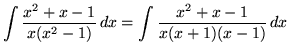(After getting a common denominator, adding fractions, and equating numerators, it follows that;

let;
let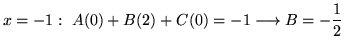;
let.)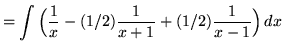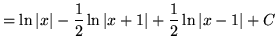(Recall that.).

SOLUTION 7 : Integrate. Decompose into partial fractions (There is a repeated linear factor !), getting(After getting a common denominator, adding fractions, and equating numerators, it follows that;
let;
let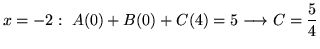;
let.)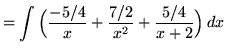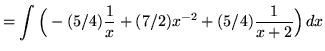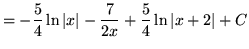.

SOLUTION 8 : Integrate. Because the degree of the numerator is not less than the degree of the denominator, we must first do polynomial division. Then factor and decompose into partial fractions (There is a repeated linear factor !), getting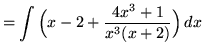(After getting a common denominator, adding fractions, and equating numerators, it follows that;

let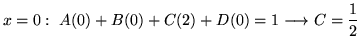;
let;
let;
let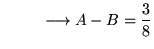;
it follows that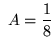and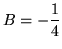.)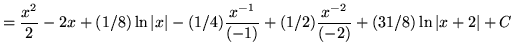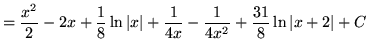.